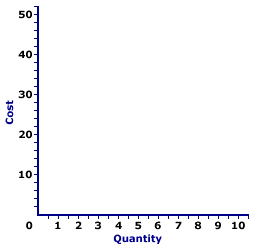Friday  December 8, 2023
 AmosWEB means Economics with a Touch of Whimsy!FINAL GOODS: Goods (or services) that are available for purchase by the ultimate or intended user with no plans for further physical transformation or as an input in the production of other goods that will be resold. Gross domestic product seeks to measure the market value of final goods. Final goods are purchased through product markets by the four basic macroeconomic sectors (household, business, government, and foreign) as consumption expenditures, investment expenditures, government purchases, and exports. Final goods, which are closely related to the term current production, should be contrasted with intermediate goods--goods (and services) that will be further processed before reaching their ultimate user.TOTAL COST CURVES:

The total cost of producing a good can be represented by three related curves, total cost curve, total variable cost curve, and total fixed cost curve. The total cost curve is the vertical summation of the total variable cost curve and the total fixed cost curve.
Total Cost CurvesThe three curves reflecting that total cost that is related to the short-run production are the total fixed cost curve, the total variable cost curve, and the total cost curve. The exhibit to the right can be used to display the three total cost curves.
• Total Fixed Cost Curve: The total fixed cost (TFC) curve is a horizontal line. Click the [TFC] button to display the curve for Wacky Willy Stuffed Amigos production. Total fixed cost is equal to \$3 and does not change with the quantity of output produced, thus the TFC curve is a flat, horizontal line.

• Total Variable Cost Curve: The total variable cost (TVC) curve is a positively-sloped line that reflects increasing then decreasing marginal returns. Click the [TVC] button to add this curve. The TVC curve emerges from the origin with a relatively steep slope, flattens, then becomes increasingly steeper. In the context of Wacky Willy Stuffed Amigos, the TVC for one Stuffed Amigo is \$5, this then rises to \$8 for two Stuffed Amigos, \$10 for three Stuffed Amigos, etc., finally reaching \$43 for ten Stuffed Amigos.

• Total Cost Curve: The total cost (TC) curve can be derived as the vertical summation of the TVC and TFC curves. In other words, the TC curve can be found by shifting the TVC vertically by the amount of TFC. This means that the shape of the TC curve is identical to that of the TVC. The two curves have identical slopes for each quantity of output. For Wacky Willy Stuffed Amigos production, the vertical difference between the TC and TVC curves is exactly \$3.00, the value of TFC. Click the [TC] button to identify this curve.
An important conclusion from this derivation of the TC curve is that the vertical distance between TC and TVC curves is the same at ALL output quantities. The reason, of course, is that this vertical distance IS total fixed cost. Because total fixed cost is constant, the vertical distance is constant.

From a geometric perspective, a constant gap between two lines means that the lines are parallel. While parallel lines are commonly presented as straight lines, two curved lines like the TC and TVC can also be parallel so long as they do not intersect and their distance remains constant.

This further implies that the slopes of the TC and TVC curves are identical at each and every output quantity. In fact, it is acceptable to think of the TC curve as the result of vertically shifting the TVC upward by the amount of total fixed cost. In other words, the TC and TVC curves are essentially the same curve, the TC curve just happens to be a little bit higher in the diagram, higher by the amount of total fixed cost.

However, in that the slope of the TC curve is marginal cost, the slope of the TVC curve is also marginal cost. Marginal cost, the increment of total cost resulting from a change in quantity, can be derived using either total cost or total variable cost. This point should be evident by noting that any quantity-induced change in total cost must come from total variable cost. Total fixed cost is fixed, it does not change. As such, marginal cost, the change in total cost, is not affected by total fixed cost.

 <= TOTAL COST CURVE TOTAL FACTOR COST =>Recommended Citation:

TOTAL COST CURVES, AmosWEB Encyclonomic WEB*pedia, http://www.AmosWEB.com, AmosWEB LLC, 2000-2023. [Accessed: December 8, 2023].

Check Out These Related Terms...

Or For A Little Background...

And For Further Study...
Search Again?GRAY SKITTERY[What's This?] Today, you are likely to spend a great deal of time looking for the new strip mall out on the highway looking to buy either a really, really exciting, action-filled video game or a coffee cup commemorating the moon landing. Be on the lookout for attractive cable television service repair people.Your Complete ScopeParker Brothers, the folks who produce the Monopoly board game, prints more Monopoly money each year than real currency printed by the U.S. government."If you don't know where you are going, any road will get you there."-- Lewis Carroll, writerATOAt The OpeningA PEDestrian's Guide Xtra CreditTell us what you think about AmosWEB. Like what you see? Have suggestions for improvements? Let us know. Click the User Feedback link.| | | | | | | | | | |
| | | |

Thanks for visiting AmosWEB